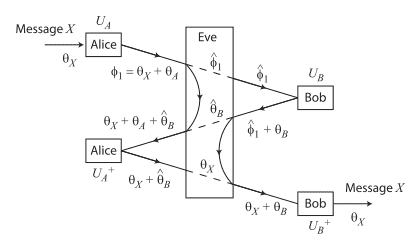# Man in the middle attack

I have some curiosity about MIM attack in a paper that I have been found before. The diagram shows the MIM attack between Alice and Bob:I found that the sentences state:

"We consider that the transmittance of the quantum channel is t. If Alice sends pulses with a mean photon number of N, Bob expects to receive pulses with mean photon number tN in the first stage and t3N in the third stage, and Alice expects to receive pulses with mean photon number t2N in the second stage. Therefore, for the MIM attack, Eve can extract a mean photon number of (1-t2)N to obtain the estimate
φ1 and a mean photon number of t(1-t2)N to obtain the estimate θB .
"

Question
How to derived (1-t2)N and t(1-t2)N?

Thank you.

#### Attachments

Mentor
Please give the reference where this is taken from.

Gold Member
•